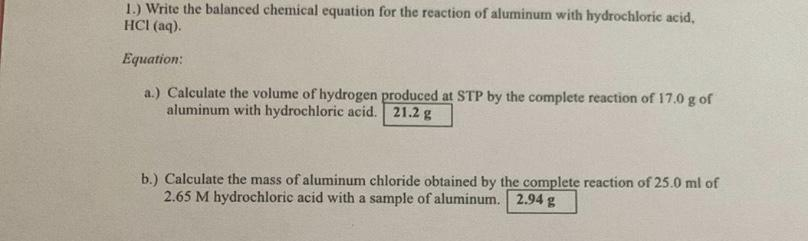Question:

# 1.) Write the balanced chemical equation for the reaction of aluminum with hydrochloric acid, HCl(aq). Equation: a.) Calculate t1.) Write the balanced chemical equation for the reaction of aluminum with hydrochloric acid, HCl(aq). Equation: a.) Calculate the volume of hydrogen produced at STP by the complete reaction of 17.0 g of aluminum with hydrochloric acid. 21.2 g b.) Calculate the mass of aluminum chloride obtained by the complete reaction of 25.0 ml of 2.65 M hydrochloric acid with a sample of aluminum. 2.94 g